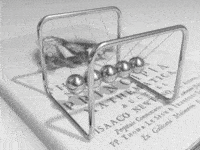# Velocity of electrons in a wire

• moment1337

#### moment1337

Electrons are moving in moves in all sorts of directions, causing the net electric charge to cancel out. But, when you apply a voltage, there's a small amount of electrons that will move towards the direction in the direction of the voltage. This is called the drift speed. Am I right? I read that the drift speed usually is 1/10000 of a second. How can you then obtain a current of 1 ampere, when 6.25*10^18 electrons has to move past a single point in one second, when the drift speed of the charge is that slow?

a small amount of electrons
There are lots of free electrons in a typical wire.

How can you then obtain a current of 1 ampere, when 6.25*10^18 electrons has to move past a single point in one second, when the drift speed of the charge is that slow?
Lots of electrons!

See: Microscopic View of Electric Current

Oh I see now. So the drift velocity of a single electron might be very slow, but because there are 8.5 * 10^28 electrons in a wire point of the wire, you still can get a pretty high current flow?

Oh I see now. So the drift velocity of a single electron might be very slow, but because there are 8.5 * 10^28 electrons in a wire point of the wire, you still can get a pretty high current flow?Have you seen this toy? It demonstrates the concept nicely. The time from an ball enters the ball chain to the end ball bouncing out is very short - but the internal balls (like the electrons in the wire) hardly move at all.

•anorlundaHave you seen this toy? It demonstrates the concept nicely. The time from an ball enters the ball chain to the end ball bouncing out is very short - but the internal balls (like the electrons in the wire) hardly move at all.

Bravo Svein. This question comes up frequently on PF. That little video you posted explains it better than 10,000 words.

Oh I see now. So the drift velocity of a single electron might be very slow, but because there are 8.5 * 10^28 electrons in a wire point of the wire, you still can get a pretty high current flow?
Exactly.